TitleCollege Algebra
Answer/Discussion to Practice Problems
Tutorial 34:Graphs of Quadratic FunctionsAnswer/Discussion to 1aVertex Note how this quadratic function is written in standard form.  That means we can find the vertex by lining it up with the general standard form and identify (h, k).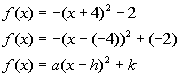*Standard form of quad. function

 Since (h, k) is the vertex in standard form, what do you think our vertex is for this problem? If you said (- 4, -2) you are correct.    Be careful about your signs on this problem.  Notice how the sign in front of h is a minus, but the one in front of k is positive.  So h is the number we are subtracting from x, which in our case is negative 4.  k is the number we are adding at the end, which our case we are adding a negative 2.

 Maximum or Minimum? Next we want to determine if the vertex that we found, (- 4, -2), is a maximum or minimum point, without graphing. If we know which direction the curve opens, that can help us answer this question.  Since a = -1, and -1 is less than 0, this parabola would open down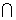. So does that mean the vertex is a maximum or minimum point? If you said a maximum point, you are right on.   So our vertex (- 4, -2) is the maximum point.Answer/Discussion to 1b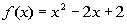Vertex Note how this quadratic function is written in the form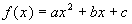.  That means we can find the vertex by using the formula.*Identify a, b, and c   *Plug values into vertex form. for a, b, and c   *Plug 1 in for x to find the y value of the vertex

 The vertex would be (1, 1).

 Maximum or Minimum? Next we want to determine if the vertex that we found, (1, 1) , is a maximum or minimum point, without graphing. If we know which direction the curve opens, that can help us answer this question.  Since a = 1, and 1 is greater than 0, this parabola would open up. So does that mean the vertex is a maximum or minimum point? If you said a minimum point, you are right on.   So our vertex (1, 1) is the minimum point.Answer/Discussion to 2aSince a = 1 and 1 > 0, then it looks like it is going to curve up. This gives us a good reference to know we are going in the right direction.

 Step 2: Find the vertex.

 Just like in problem 1a above, this quadratic function is written in standard form.  That means we can find the vertex by lining it up with the general standard form and identify (h, k).*Standard form of quad. function

 Since (h, k) is the vertex in standard form, what do you think our vertex is? If you said (-2, 1) you are correct.  Be careful about your signs on this problem.   Notice how the sign in front of h is a minus, but the one in front of k is positive.  So h is the number we are subtracting from x, which in our case is -2.  k is the number we are adding at the end, which our case we are adding a 1.

 y-intercept Reminder that the y-intercept is always where the graph crosses the y-axis which means x = 0:*Replace x with 0

 The y-intercept is (0, 5).   x-intercept Reminder that the x-intercept is always where the graph crosses the x-axis which means y = 0: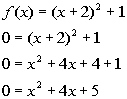*Replace y (or f(x)) with 0

 Note that this does not factor.  Let's try solving by using the quadratic formula: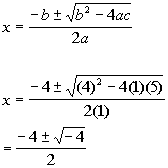*Plug in values for a, b, and c

 Note how we got a negative number underneath the square root.  That means there is no real number solution.  That also means that there are NO x-intercepts.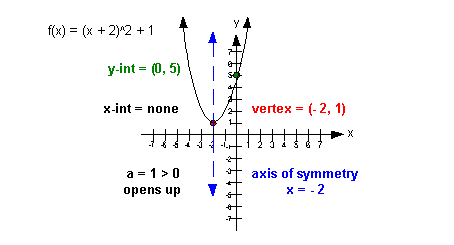Axis of symmetry As shown on the graph, the axis of symmetry is x = -2.Answer/Discussion to 2b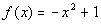Since a = -1 and -1 < 0, then it looks like it is going to curve down. This gives us a good reference to know we are going in the right direction.

 Step 2: Find the vertex.

 Just like in problem 1b above,  this quadratic function is written in the form.  That means we can find the vertex by using the formula.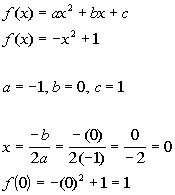*Identify a, b, and c   *Plug values into vertex form. for a, b, and c   *Plug 0 in for x to find the y value of the vertex

 So the vertex is (0, 1).

 y-intercept Reminder that the y-intercept is always where the graph crosses the y-axis which means x = 0:*Replace x with 0

 The y-intercept is (0, 1).   x-intercept Reminder that the x-intercept is always where the graph crosses the x-axis which means y = 0: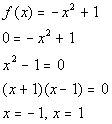*Replace y (or f(x)) with 0 *Solve the quadratic by factoring

 The x-intercepts are (-1, 0) and (1, 0).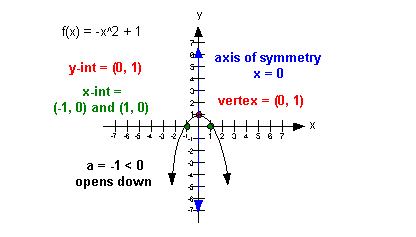Axis of symmetry As shown on the graph, the axis of symmetry is x = 0.

Last revised on July 10, 2010 by Kim Seward.
All contents copyright (C) 2002 - 2010, WTAMU and Kim Seward. All rights reserved.